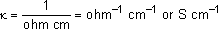Electrolytic Conductance, Molar Conductance And Specific Conductance for JEE Mains

IIT JEE Chemistry Factors On Which The Conductance Depends

CONDUCTANCE:-

Conductance is defined as a degree to which an object or a conductor can conduct the electric current through it. The conductance is a property which is labeled as G. Conductance is the reciprocal of resistance as it is calculated as a ratio of current ‘I passing to the potential difference ‘V. Ohms law is used to define the conductance. The unit of conductance is Siemens (S) or mho. Therefore, the conductance G = I/V=1/R mho or S. Not only in electronics but conductivity measurements are also done in ecology and environmental science to the level of nutrients in lakes and rivers.

The factors on which the conductance depends upon are as follows:

• 1) Length of the conductor.

• When the length of the conductor increases, the resistance increases. Hence the resistance is directly proportional to the length of the conductor and is inversely proportional to conductivity.
R α l where l is the length of the conductor

• 2) Area of cross-section of the conductor.

• The area of cross-section is inversely proportional to the resistance.
R α 1/A where A is area of cross-section

• a) Conductivity offers a fast, reliable, nondestructive, inexpensive and durable means of measuring the ionic content of a sample.

• b) Reliability and repeatability are excellent.

• Disvantages:-

• a) The main drawback of conductivity is that it is a nonspecific measurement; that is, it cannot distinguish between different types of ions, thereby giving instead a reading proportional to the combined effect of all ions present. Therefore, it must be applied with some pre-knowledge of the solution composition or used in relatively pure (single solute) solutions to be successful.

• ELECTROLYTIC CONDUCTANCE:-

Electrolytic conductance is defined as the flow of electricity through an electrolytic conductor. The electrolytic conductor is a conductor through which the electricity passes in the form of ions. When a conductor is melted or the temperature is increased at one end then the dissociation of electrolyte takes place in the form positive ions and negative ions. In case of electrolytes, the conductor is an electrolytic solution and hence its ability to conduct ions through it is measured. The conductance of an electrolyte is calculated by measuring the resistance between the two nodes. When heat or electricity passes through an electrolyte, the solution dissociates into cat-ions, that is, positive ions and anions that is negative ions. The conduction in electrolytes takes place when the electrolytes are in their liquid form. Some factors that affect the conductance in electrolytes such as temperature, viscosity also depends upon how much concentrated the ions are.

The nature of the electrolyte also plays the important role in the conductance. The size of the ions produced and their salvation also affects the conductivity of an electrolytic solution. The number of ions in the electrolyte decides the nature of an electrolyte solution that is, if an electrolyte is a weak electrolyte it means that the number of ions in the solution are less. If the electrolyte is weak then the conduction will also be weak. Stronger the concentration, the greater will be the conductance. Similarly the temperature is an important parameter. The temperature helps to dissolve an electrolyte and hence enhances the degree of dissociation and automatically the conduction. The electrolytic conductivity also depends upon the mobility of the ions. The attraction between the ions decreases with an increase in the temperature which in turn increases the mobility and the ions in the electrolytes gain energy which increases their velocity and hence the conductivity.

The following figure shows how the conductance of the electrolyte is obtained using the galvanic cell or by the method of electrolysis.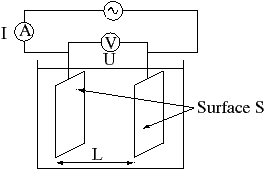The two plates placed as electrodes in the solution and are connected with the help of voltmeter and the AC current is supplied so that electrolysis does not take place.

The electrolyte conductance is achieved by calculating the two plates separated at distance l as shown in figure. This device is called as Electrical Conductivity meter. It is mostly used in the laboratories to monitor the impurity, salt nutrients in the water.
The basic working principle of this meter is the potentiometric method. Platinum metal is usually used as an electrode in the electric conductance meter.

Even though the conductivity can be obtained using the distance and surface area of the electrodes, but for reliability the calibration of electrolyte is done.

MOLAR CONDUCTANCE:-

The conductivity of an electrolyte divided by the molar concentration is said to be the molar conductance.

The molar conductivity is labeled as ‘λ’. Molarity of the electrolyte is denoted as M. Therefore, the molar conductivity of an electrolyte dissolved in one molar of its solution can be calculated by the following formula:
λ= k/M …… k is specific conductance.

As the electrolytic conductivity is not suitable for comparing the conductance of different solution as every solution contains different number of ions in different concentration. So, the concept of the Molar conductivity is used to determine the conductance of the solution.

Unlike electrolyte conductivity, the molar conductivity also varies according to the nature of the electrolytes. But in this case more the electrolyte is diluted, the more number of moles start to dissociate. Hence, the Molar conductance increases. The weak electrolytes have less molar conductance. The molar conductivity of the strong electrolytes increases when the electrolyte starts to dilute.

When the solution gets diluted, the mobility of the ions increases as the viscous value is decreased.

The following equations will help us to define the Molar conductance more accurately.

Lets’ consider the specific conductivity ‘k’ of the one mole of electrolyte diluted in solution V. Hence, the relation between molar conductance and specific conductance can be given as follows

Molar conductivity = specific conductivity * V
λ = k* V

Therefore, the units of molar conductivity will be ohm–1 cm2mol–1 or S cm2mol–1.

According to the Friedrith Kohlrausch experiment, the Molar conductivity of strong electrolyte varies linearly with square root of the concentration.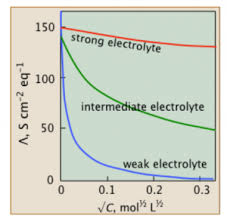The above graph shows the relation between the molar conductance and the nature of the electrolytes. We know that the electrolytes are of three types depending on the concentration of ions, that is, strong electrolyte, weak electrolyte and intermediate electrolyte.

The electrolytes are called strong electrolytes if the ions in the electrolyte completely dissociates in the solution when in aqueous form. The ions in these electrolytes are very good conductors of electricity. Hence, when the dilution increases the molar conductivity of the electrolyte also increases. Therefore, the graph shows the straight line showing the linearity with y-axis.

The electrolytes will be called as weak electrolyte if the ions in the electrolyte partially dissociate in the solution. The weak electrolytes in comparison with the strong electrolytes have the lower degree of dissociation hence get ionized partially and therefore show a drastic change in deviation. Therefore, the limiting molar conductance cannot be obtained for the weak electrolytes.

SPECIFIC CONDUCTANCE:-

Ability of an electrolyte to conduct electricity in meters is called as specific conductance. The specific conductance is measured according to the length and the area covered by an electrolyte or the conductor. It is also inversely proportional to specific resistance ‘ρ’. It is given as Siemens/meter or G/m. it is denoted by ‘k’.

Where we say,
R α 1/A, where A is area of cross section,
R α l, where l is the length of the conductor,
R = ρ l/A ----- (1) where ρ is constant of proportionality called as specific resistivity.

Thus, we come to know that the resistivity of the material is constant and only depends on the nature of the material. Let us take an example of two copper wires, one is 1 cm and other is 3 cm long. Both the copper wires have constant resistivity. So, the resistivity depends only on the nature of the material. The reciprocal of specific resistance is called as specific conductance kappa ‘k’. Le us consider the specific conductance ‘k’ and the conductance ‘G’.

Then R= 1 / G and ρ = 1 / k

Therefore, from the above equation (1), we get that
1 / G = 1/k* l / A

Therefore, the specific conductance ‘k’ becomes
k = G l / A ----- (2) i.e.

Specific conductance = Conductance X Cell Constant.

From the above equation we come to know that l/A is characteristic of the cell used to measure the conductance. This characteristic constant of the cell is called as cell constant.

Let’s consider an example such that the distance between the two electrodes is 1 cm and the area of cross-section A is 1 cm
Now l = 1 cm
A = 1 cm square then according to equation (2);
k = G l / A we get
k = G 1cm / 1 cm2

Therefore, k = G.

Thus, the conductance of two electrodes, 1 cm apart with cross-section of 1cm square can be defined as specific conductance.
Example of the cube below also defines the specific conductance,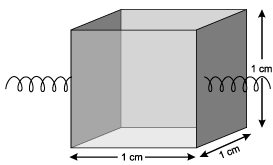The specific conductance of the cube is equal to conductance.

We can also get the units of the specific conductance as follows
Resistivity ρ = RA / l

That is,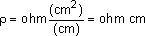Conductivity k =1/ρ,